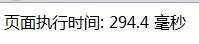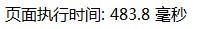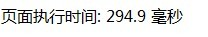# php中使用in_array() foreach array_search() 查找数组是否包含时的性能对比

更新时间：2015年04月14日 22:39:49   投稿：mdxy-dxy我要评论

```<?php
\$runtime= new runtime;
\$runtime->start();
\$a = 'k';
\$b = array('a','b','c','d','e','f','g','h','i','j','k');

/*
for (\$i=0; \$i < 100000; \$i++) {
var_dump(in_array(\$a, \$b));
}
*/

/*
for (\$i=0; \$i < 100000; \$i++) {
foreach (\$b as \$key => \$value) {
if (\$a == \$value) {
//echo TRUE;
continue;
}
}
}
*/

/*
for (\$i=0; \$i < 100000; \$i++) {
array_search(\$a, \$b);
}
*/

\$runtime->stop();
echo \$_b;
echo "执行时间: ".\$runtime->spent()." 毫秒";

class runtime{
var \$StartTime = 0;
var \$StopTime = 0;
function get_microtime(){
list(\$usec, \$sec) = explode(' ', microtime());
return ((float)\$usec + (float)\$sec);
}
function start(){
\$this->StartTime = \$this->get_microtime();
}
function stop(){
\$this->StopTime = \$this->get_microtime();
}
function spent(){
return round((\$this->StopTime - \$this->StartTime) * 1000, 1);
}
}
?>```

in_array()foreacharray_search()